﻿ An Experimental Investigation of Long-Range Projectiles under Different Wind ConditionsPublications are Open
Access in this journal
Article Versions
Export Article
• Normal Style
• MLA Style
• APA Style
• Chicago Style
Original Article
Open Access Peer-reviewed

### An Experimental Investigation of Long-Range Projectiles under Different Wind Conditions

M. Nasir Uddin, Md. Humayun Kabir Bhuiyan, Dr. Golam Mostofa
American Journal of Mechanical Engineering. 2021, 9(1), 7-17. DOI: 10.12691/ajme-9-1-2
Received November 21, 2020; Revised December 25, 2020; Accepted January 08, 2021

### Abstract

Due to the rapid development of the Field Artillery Weapon System (AWS) the projectiles play an important role in modern time ammunition and the development of an efficient projectile is very important. The design parameters of a projectile depend on the drag and lift force acting on it. Therefore, a detailed experimental and simulation is required to understand the projectile performance against the wind. In this article, an experimental and numerical investigation of long-range Artillery projectile (Field and Medium) under different wind conditions are carried out. Three different sizes of projectiles are used in this experiment (105, 122, and 130 mm). The experiment is carried out by placing the projectile in front of the wind tunnel. The simulation was done using simulation software packages changing the Angle of Attack (AOA) (30°, 35°, 40°, 45°, and 50°) keeping the wind velocity and geometry the same. The pressure coefficients have been calculated from the measured values of the surface static pressure distribution on the projectile. Later, the drag and lift coefficients are obtained from the measured pressure and a projected area of the projectiles. The wind flow effect on the projectiles is also analyzed by Ansys software. The simulation and experimental results show a similar trend regarding, drag forces and lift forces. The simulation result shows that the size of the projectile is an important factor that is mostly related to the drag and as well as lift forces.

### 1. Introduction

Due to the large use of long-range projectiles worldwide, the study of aerodynamic characteristics of projectiles has become one of the most important focuses for its improvement. Within the field of projectile design, there has been a frequent endeavoring to increase the range and precision target hitting of projectiles 1, 2, 3. The increased range is achieved by the performance improvement of the projectiles. However, the forecast of drag coefficients and lift coefficients for diverse formations are crucial. However, various parameters such as fins and jets can be utilized to increase aerodynamic characteristics for better control on maneuvering projectile. Hence, several research works have been carried out by using various smart technology to improve the performance of the projectiles 4, 5, 6. Self-adaptive ballistic correction is considered an important parameter nowadays by applying numerous advanced intelligent control 6. Besides, stability and optimization of projectiles are the significant factors for the flight performance by reducing drag 7, 8. They have noted that inadequate system stability outcomes in the failure of operations.

Moreover, the long ranges and precise targets hitting by a projectile are projected to be persistently enriched, particularly when a new projectile is developed or an existing projectile is modified for upgraded 8. Consecutively, the hollow projectile known as a tubular projectile is an important parameter to be considered to improve its performance characteristics greatly 9. Research on optimal hollow projectile shows that the bow shock wave in front of it results in the projectile drag coefficient reductions abruptly. Ever-increasing demands for accuracy and range in modern warfare have expedited the optimization of a projectile design. The crux of projectile design lies in the understanding of its aerodynamic properties early in the design phase.

Therefore, studying the projectile based on their sizes, shapes, air velocity and Angle of Attack of the air stream seems an important parameter to optimize the design of a projectile. This study forecasts the investigation of projectiles under different wind conditions. The demonstration is being performed to investigate the effect of design parameters on the system performance of the projectile and its effective flight path.

1.1. Motivation

Smart, intelligent, and high mobility of ammunition is the important development direction of ammunition technology in a long historical period. In the future, self-adaptive ballistic correction and autonomous attack smart ammunition by applying various innovative intelligent control technology have become the research focus of the world's national defense science and technology.

One of the most important aerodynamic performance characteristics for the projectile is the total drag. The total drag for projectiles can be divided into three components: (i) pressure drag (excluding the base), (ii) viscous (skin friction) drag, and (iii) base drag. The base drag is a major contributor to the total drag, particularly at the transonic speeds. Thus, the minimization of base drag is essential in minimizing the total drag of the projectiles. The breakdown of the total drag into various components is important in the preliminary design stage of a projectile. This information can aid the designer to find potential areas for drag reduction and achieve the desired increase in range and/or terminal velocity of projectiles since they are affected by the projectile drag.

Due to the rapid development of the Field Artillery Weapon system over time, many researchers have been excited by the wind. In the present study, the effect of static loading is taken into account due to the steady wind. Since natural winds are continually fluctuating, it is generally assumed that these fluctuations are so irregular and random that the response of a structure will not differ from that due to a steady wind of the same average speed.

1.2. The Effect of Wind on Projectiles

The effect of wind on the projectiles as a whole is determined by the combined action of external and internal pressures acting upon it. In all cases, the calculated wind loads act normal to the surface. The pressures created inside a hollow shape projectile due to access to wind through openings could be suction (negative) or pressure (positive) of the same order of intensity while those outsides may also vary in magnitude with possible reversals. Thus, the design value shall be taken as the algebraic sum of the two inappropriate/concerned direction. Furthermore, the external pressures (or forces) acting on different parts of a projectile do not correlate fully. Hence, there is a reduction in the overall effect.

The development of modern materials and construction techniques has resulted in the emergence of a new generation of projectiles. Such projectiles exhibit increased susceptibility to the action of wind. Accordingly, it has become necessary to develop tools enabling the designer to estimate wind effects with a higher degree of refinement. It is the task of the engineer to ensure that the performance of projectiles subjected to the action of wind will be adequate during their anticipated flight path from the standpoint. To achieve this, the designer needs information regarding (i) the wind environment, (ii) the relation between that environment and the forces it induces on the structures, and (iii) the behavior of the projectiles under the action of forces.

The flow around projectiles in the actual environment is very complex and formulation of a mathematical model to predict the flow is almost impossible. Thus, the model study is a must and the results obtained under the simulated condition in the laboratory are found to be quite satisfactory for practical purposes.

1.3. Objectives

In the present experimental investigation different types of hollow shape artillery projectile like 105 mm, 122 mm, and 130 mm have been taken into consideration. The objectives of the research are as follows:

a. To measure the lift and drag force of projectiles depending on the size, shape, and angle of attack by using a subsonic wind tunnel.

b. Numerical modeling of lift and drag coefficient for wind flow over the projectiles.

c. Validation of numerical modeling using experimental data.

The results will be expressed in non-dimensional parameters, so that it may be applied for different types of prototype projectiles. The findings will enable the engineers to design different special projectiles effectively.

### 2. Experimental Setup

The research has been carried out based on a theoretical analysis for a small scale projectile along with the formulation of the mathematical model and numerical analysis. The mathematical model is to be formulated by understanding the wind nature, analyzing the mechanics of projectile-wind interaction, and the interaction of air-flow-aerodynamics. The selected projectile is to be then used for simulating the system performance, optimizing the design parameters, and effective range. Then the optimized design parameters will be used for developing a numerical model and relationship between lift coefficients and drag coefficients with free stream velocities and angle of attacks with the help of ANSYS software.

2.1. Experimental Conditions

There were three Artillery projectiles to be tested at different Angle of Attack (AOA). The number of tapping points around the projectiles is the same for all three projectiles. The static pressure is measured at each tapping point with an inclined manometer. The detailed experimental conditions are shown in Table 1. The CFD simulation was done on similar conditions to compare the experimental and simulation results.

In each case of the tests, wind velocity is measured directly with the help of a digital anemometer. The flow velocity in the test section 4.7 m/s approximately. The measured velocity distribution was almost uniform across the tunnel test section on the upstream side of the test models.

The projectiles were placed with a stand at the same level as the wind tunnel at the exit end. In the middle of the hollow cylinder, it was made groove and connected with a plastic tube. Either side of the plastic tube is connected with an inclined multi-manometer. Each circular projectile was equally spaced and made a total of 30 taps. Each manometer is made with 30 tubes and connected with projectile grooves. There were 30 scales fixed along the 30 tubes in the manometer to take the reading. The projectiles were placed at the exit end of the wind tunnel first at a 30° angle of elevation. Then it was gradually elevated at the interval of 5° each and data is recorded. In this way, projectiles were elevated up to (30°,35°,40°,45°, and 50°) and necessary data was recorded and subsequently, calculations are carried out.

2.2. Test Setup

The experiment has been conducted in an open circuit subsonic wind tunnel installed at Mechanical Fluid Mechanics Laboratory in MIST. The successive sections of the wind tunnel comprise a bell mouth entry, a flow straightener, a diverging section, and two axial flow fans. Flow along the central longitudinal axis of the wind tunnel was maintained at a constant height from the floor. The hollow Artillery projectiles were placed at the exit end of the wind tunnel. A set of hollow shape Artillery projectiles (105, 122, and 130 mm) have been developed for the experiment. Here the static pressure measurement of hollow shape Artillery projectiles has been done at various angles of attack. Finally, the performance parameters of the developed projectile models have been investigated experimentally and numerically.

• Figure 1. Schematic diagram of the wind tunnel used in the experiment

Each of the fans is connected with a motor of 2.25 kilowatt and 2900 rpm. There is a regulator to control the wind speed. There is a silencer as shown in Figure 1 to control the noise. The converging mouth entry is incorporated in the wind tunnel for smooth entry of air into the tunnel and to maintain uniform flow into the duct-free from outside disturbances. The induced flow through the wind tunnel is produced by a one-stage rotating axial flow fan of capacity 10.20 m3/s at 1475 rpm. The diverging and converging section of the wind tunnel is 965 mm long and made of 18 GMS black sheets. The angle of divergence and convergence is 29°, which has been done to minimize expansion and contraction loss.

2.3. Preparation of the Projectiles

For this experiment, specimens of 105 mm, 122 mm, and 130 mm Artillery projectiles have been prepared. Each of the projectiles was made of seasoned teak wood to avoid buckling and expansion due to the change of temperature and humidity. The 105 mm projectile is shown in Figure 2(a) and the tapping position is shown in Figure 2(b). Each tapping was identified by a numerical number from 1 to 30 for each of 105 mm, 122 mm, and 130 mm Artillery projectiles. The tappings were made along the circular section of the projectiles. The projectile was hollow inside through which the plastic tubes were allowed to pass. The plastic tubes were connected with the copper tubes at one side and the other side with the inclined multi-manometer shown in Figure 3.

• Figure 2. (a) 105 mm Projectile and (b) the tapping points on it
• Figure 3. Position of the projectiles against the wind and measurement of the pressure at the tapping points of the projectile. (Fluid Mechanics Lab of MIST)

### 3. Mathematical Model and Simulation

3.1. Mathematical Model

The calculation procedure of finding pressure coefficients, drag and lift coefficients has been described briefly in this section along with the simulation procedure. Then, the static pressure on the projectile surface is measured. The air velocity of the air stream is obtained from the anemometer. The projected area of the segmented part of the projectiles is calculated through the SolidWorks model. The drag (CD), lift (CL), and pressure coefficient (CP) are calculated from the static pressure, air velocity, and projected area. The design of the projectiles was done through reverse engineering of the real long-range projectiles used in the real battlefield. The projectiles are manufactured with seasoned teak wood material in a lathe machine. The holes are made on the surface of the projectiles to measure the pressure acting on them. Figure 4 shows the manufactured projectiles with wood.

• Figure 4. Wooden projectiles manufactured

The projectiles are segmented into 30 strips and then a 3D drawing was drawn to connect the top and bottom of the projectiles. Then, a 2D drawing was drawn on a plane facing the air stream. The area of all segments was not calculated as they can not be seen from the front of the plane facing the wind. Therefore, the pressure difference in those areas is fluctuating due to turbulence and not dependable. Figure 5, Figure 6, and Figure 7 show the projected area measurement by SolidWorks.

• Figure 5. Plane view of the Projectile form air direction
• Figure 6. The area has drawn from the projection of the segments
• Figure 7. Measurement of the projected area

The pressure at the tapping is measured by using Equation 1.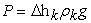(1)

Where

P= Pressure

ρk = Density of Kerosene

g= Gravitational Acceleration.

The acting force on a single segment (assuming segment 1) is calculated from Equation 2.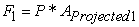(2)

Then the total force acting on the Projectiles will be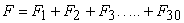(3)

As the air is coming at an angle, therefore, the total forces will be divided into Horizontal and Vertical directions. If the Angle of Attack is ‘α’ then the drag and lift force is calculated from Equations 4 and 5.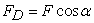(4)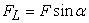(5)

The Drag Coefficient (CD), Lift Coefficient (CL), and Pressure Coefficient (CP) are calculated from Equation 6, 7, and 8.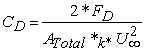(6)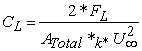(7)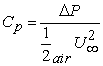(8)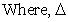P = P – P0

P = Static pressure on the surface of the Projectile.

P0 = The ambient pressure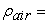The density of the air

U= The free stream velocity

ATotal= Total Active Projected Area (A1+A2+A3+………+An).

3.2. Computational Flow Simulation

Computational fluid simulation is done on all the projectile according to the experimental conditions. Ansys software is used to analyze the CFD model. The SolidWorks model made for measuring the projected area is used for simulation. The projectile is considered as a solid domain and outside of it is considered as air domain. The k-ε turbulence model is used for solving the problem. The inlet condition was 4.7 m/s air velocity and the outlet condition was atmospheric condition similar to the experiment. The rest of the surface is considered a wall. Figure 8 shows the geometry of the 105 mm projectile, and the mesh file for simulation of the same projectile is shown in Figure 9.

• Figure 8. Geometry files for CFD simulation of 105 mm projectile
• Figure 9. Mesh of the CFD simulation for 105 mm projectile

The geometry of the projectiles with the same dimension was put forward to simulate with scale 1:1. The geometric model of a projectile is shown in Figure 8. The projectile model was sketched on the SolidWorks 2017 then imported to ANSYS Geometry Module where the computational domain for the model is created as shown in Figure 8. The boundaries are chosen at the front, right, left, top, and rear up to 10D, 10D, 10D, 10D, and 15D respectively from the surface of the model where D is the downstream radius of the projectile.

In the simulation, the platform of pre-processing ICEM, CFD 16.0, unstructured grid was adopted. After that sphere of influence, an approach was employed to densify the gird around the model to improve the numerical precision, as shown in Figure 9. In Figure 9, the mesh generated is 2143025 elements, 2871030 nodes. The results obtained during the grid dependency tests a finer mesh, made of 2143025 elements, 2871030 numbers of nodes are compared with a coarse mesh of 192994 elements and 64138 numbers of nodes. The pressure coefficient found for the finer mesh varies with the coarse mesh by 0.57% which is concurrent to the independency test.

The air enters the domain with a velocity of 4.7 m/s. The density of air was 1.225 kg/m3 and viscosity about 1.7894e-05 kg/m-s. At the outlet, the pressure outlet condition is applied to the domain. The steady and incompressible flow of air is considered in this Analysis. The solution procedure was adopted to solve the CFD model using the FVM solver. The pressure-based solver is used for solving the steady-state problem. Atmospheric pressure is maintained at the outlet therefore use the default value (‘0’ Pa for gauge pressure). In these calculations, the second-order upwind scheme based on the multidimensional linear reconstruction approach is used. The SIMPLE algorithm for pressure velocity coupling with a second-order upwind discretization scheme is used to obtain a solution for the equations of Momentum, Turbulence Kinetic Energy, and Turbulence Dissipation Rate. The target of all discretization techniques in FVM (Finite Volume Method) is to develop a mathematical model to convert each of the terms into an algebraic equation. Once implemented to complete control volumes in a particular mesh, we attain a full linear system of equations that requires to be solved. These computations are carried out using FVM solver (ANSYS FLUENT 2016), a commercial CFD package with a 3D double-precision configuration. The default convergence criterion in FLUENT is maintained. This criterion requires that the scaled residuals decrease to 10-4.

### 4. Results and Discussion

The manometer reading was converted into pressure and the total force was calculated based on the segmented area of the projectile. The drag force and lift forces were calculated based on the angle of attack. The total drag force and lift force was summed up for the whole projectile and later the lift, drag, and pressure coefficients were calculated. The experimental data shows that the drag and lift forces are increasing with the increase in the AOA and projectile size.

The simulation data shows that the drag force and lift force is increasing as the angle of attack is increasing for constant air velocity. However, the drag forces and lift forces are slightly lower at 45 angle of attack. The drag and lift forces are increasing as the size of the projectiles is increasing. The simulation result shows a similar trend as an experiment where the drag and lift force is increasing with the increased size of the projectile. Figure 10 shows the comparison of the simulated and experimental drag and lift forces at a different angle of attack (AOA). The data for the corresponding plots are shown in Table 2 and Table 3.

• Figure 10. (a) Drag Force (b) Lift Force at different Angle of Attack (Experiment vs Simulation)

The Drag and Lift Coefficients are calculated from simulation and experiment. The experimental drag and lift coefficients were mostly higher than the simulation. This may be due to the surface roughness of the specimen and minor geometrical inaccuracies as the specimen was manufactured on a manual Lathe Machine. However, the Drag and lift coefficients for the 105 mm projectile was found mostly lower than the simulation except for the lift coefficient at 50 angle where it was found higher than the simulation result. This could be the effect of the projectile shape as the shape (the shape is changed as AOA is changed) of the projectile play an important role in the aerodynamic characteristics. The drag and lift coefficient for the same projectile increased little and did not increase much as the AOA attack increased from 30 to 50. Similar phenomena were found 10 where the drag coefficient is increased until Mach 1.1-1.20 and after that, the drag coefficient has started to reduce shown in Figure 11. However, initially, the drag coefficient seems to increase linearly in subsonic air velocity. Another study 11 has found from the simulation that, the Drag Coefficient has increased as the AOA increased which is found in our case as well. Figure 12 shows the experimental and simulated drag and lift coefficient plot at different angles of attack and Table 4 and Table 5 shows the corresponding data for drag and lift coefficients.

• Figure 11. Dependence of the drag coefficient of the projectile on the air velocity
• Figure 12. (a) Drag Coefficient (b) Lift Coefficient at different Angle of Attack (Experiment vs Simulation)
• Figure 13. Tapping point Vs pressure coefficients at different Angle of Attack (AOA) for 105 mm projectile
• Figure 14. Tapping point Vs pressure coefficients at different Angle of Attack (AOA) for 122 mm projectile
• Figure 15. Tapping point Vs pressure coefficients at different Angle of Attack (AOA) for 130 mm projectile

The pressure coefficient is calculated and plotted against the tapping points on the projectiles. The pressure coefficients at the tapping points that are facing the air gradually decreasing and increasing. The measurement at the back of the projectile is very fluctuating as turbulence was observed in the back. Therefore, the pressure coefficients at the back of the projectile are not dependable. It was also observed that the turbulence felt at the back of the projectile is related to the size of the projectile. The turbulence decreased as the projectile size increased from 105 mm to 130 mm shown in Figure 13, Figure 14, and Figure 15.

• Figure 16. The pressure contour for 105 mm projectile at 45AOA
• Figure 17. The velocity contour for 105 mm projectile at 45AOA
• Figure 19. The velocity contour for 122 mm projectile at 45AOA
• Figure 21. The velocity contour for 130 mm projectile at 45AOA

The simulation pressure and velocity gradient are shown for 105 mm, 122 mm, and 130 mm projectiles in Figure 16, Figure 17, Figure 18, Figure 19, Figure 20, and Figure 21. The pressure contour shows that the pressure is more felt at the front of the projectiles for all sizes. However, the velocity streamline plot shows that the streamline is flowing over the 105mm projectile. The 122 mm and 130 mm projectiles do not show any streamline flowing over them. Therefore, the drag forces should be higher for larger projectiles.

The simulation reveals that there is almost no air streamline is flowing over the 122 mm and 130 mm projectiles that may increase the drag forces significantly. The pressure contour shows more pressure at the front of the projectiles that come in contact with the air directly. Again, the pressure at the back of the projectiles only comes in contact with the air due to the turbulence. Therefore, the pressure is found negative and the manometer felt suction while the pressure coefficient shows more turbulence for the smaller size projectiles. The experimental and simulation results show some diversion which may result from many sources that need to address in future studies.

### 5. Conclusions

In this article, an experimental and simulation investigation was performed on the projectiles model designed from the existing artillery projectile by reverse engineering. The experiment was carried out with a subsonic wind tunnel and similar experimental conditions were applied for numerical simulation to investigate the further parameters that are not possible to measure or visualize in real-time. However, the conclusions are drawn from this study are as follows:

a. The simulation and experimental results found in this investigation show that the drag force and lift forces are increasing with the increasing angles of attack.

b. The drag and lift force also increases as the size of the projectile is increased.

c. The trend for increasing the drag forces are smaller at a lower angle and gradually increases with increasing angle of attack. The simulation and experimental results show a similar trend for drag forces.

d. The study found that the lift force increasing rate is lower than that of drag forces with the increasing angle of attack.

e. The drag forces and lift forces acting on the projectiles are a combined effect of the size, shape, and angle of attack the front of the projectiles that come in contact with the air directly.

f. The pressure at the back of the projectiles only comes in contact with the air due to the turbulence. Therefore, the pressure is found negative and the manometer felt suction while the pressure coefficient shows more turbulence for the smaller size projectiles.

The experimental and simulation results show some diversion which may result from many sources that need to address in future studies. This CFD model can be used to study the projectiles in supersonic speed to investigate more details considering supersonic boundary conditions such as compressibility of air.

### Acknowledgments

The authors would like to give thanks to the Military Institute of Science and Technology (MIST) and the Workshop Division and Fluid Mechanics Lab of the Department of Mechanical Engineering, MIST for providing financial support and laboratory facilities.

### References

  Bolonkin, A., “Long distance bullets and shells,” International Journal of Aerospace Sciences, 2(2). 29-36. 2013. In article  Suliman, M.A., Mahmoud, O.K., Al-Sanabawy, M.A., Abdel-Hamid, O.E., ‘‘Computational investigation of base drag reduction for a projectile at different flight regimes,’’ 13th International Conference on Aerospace Sciences & Aviation Technology, Paper ASAT-13-FM-05, 1-13. 2009. In article View Article  Sahu, J., (2003), “Unsteady numerical simulations of subsonic flow over a projectile with jet interaction,” 41st Aerospare Sciences Meeting and Exhibit, AIAA 2003-1352, 1-10. 2003. In article View Article  Sahoo, S., Laha, M.K., (2014), “Coefficient of Drag and Trajectory Simulation of 130 mm Supersonic Artillery Shell with Recovery Plug or Fuze,” Defence Science Journal, 64 (6). 502-508. 2014. In article View Article  Novak, L., Bajcar, T., Širok, B., Orbanić, A., Bizjan, B., “Investigation of Vortex Shedding from an Airfoil by Computational Fluid Dynamic Simulation and Computer-Aided Flow Visualization,” Thermal Science, 22 (6B), 3023-3033. 2018. In article View Article  Wessam, M.E., Huang, Z., Chen, Z., “Aerodynamic characteristics and flow field investigations of an optimal hollow projectile,” Proceedings of the 5th International Conference on Mechanical Engineering and Mechanics, 181-186. 2014. In article  Yongjie, X., Zhijun, W., Guodong, W., Jianya, Y., Shouli, P., “Ballistic characteristics of rocket projectile with deflection nose,” International Power, Electronics and Materials Engineering Conference (IPEMEC 2015), 405-411. 2015. In article  Lijin, J., Jothi, T.J.S., (2018), “Aerodynamic Characteristics of an Ogive-nose Spinning Projectile,” Sadhana, 43(63). 1-8. 2018. In article View Article  Dali, M.A., Jaramaz, S., “Optimization of Artillery Projectiles base Drag Reduction using Hot Base Flow”, Thermal Science, 23 (1). 353-364. 2019. In article View Article  Alexey. M.L, Stanishlav, A.K, Ivan. G.R, (2017), “Optimization of Aerodynamic Form of Projectiles for Solving the Problem of Shooting Range Increasing”, AIP Conference Proceeding 1893, 030085. In article View Article  A. Hemateja., B. Ravi Teja., A, Dileep Kumar., Rakesh, S.G., “Influence of Nose Radius of Blunt Cones on Drag in Supersonic and Hypersonic Flows”, Material Science and Engineering, 225 (2017) 012045. In article View Article

Published with license by Science and Education Publishing, Copyright © 2021 M. Nasir Uddin, Md. Humayun Kabir Bhuiyan and Dr. Golam MostofaThis work is licensed under a Creative Commons Attribution 4.0 International License. To view a copy of this license, visit http://creativecommons.org/licenses/by/4.0/